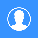مدرس اون لاين

# Work - Kinetic Energy - Potential Energy - Conservative Forces - Conservation of Mechanical Energy - Newton's Law of Universal Gravitation

## 12022012Work - Kinetic Energy - Potential Energy - Conservative Forces - Conservation of Mechanical Energy - Newton's Law of Universal Gravitation

Work - Kinetic Energy - Potential Energy - Conservative Forces - Conservation of Mechanical Energy - Newton's Law of Universal Gravitation
http://videolectures.net/mit801f99_lewin_lec11/
1. 1D Work and Kinetic Energy:

The equation for work, and its units are introduced. The work-energy
theorem is derived showing that the change in kinetic energy equals the
work done on a particle by the sum of all forces (thus the net force).
Gravity does negative work on an object thrown upwards until it reaches
its maximum height of its trajectory.

2. Work Calculated in 3-Dimensions:

Work in 3D is shown to decompose into the sum of each 1D component.

3. Gravity is a Conservative Force:

Work done by gravity while a particle moves upwards a vertical
distance h is -mgh, regardless of the path taken. When the work done by a
force is independent of the path, that force is called a conservative
force.

4. When Gravity is the only Force:

The equation for gravitational potential energy is introduced by
rearranging the work-energy theorem. Potential energy and kinetic energy
can be converted back and forth but their sum, the mechanical energy,
is conserved if only conservative forces are involved. Friction is not a
conservative force. When friction is at stake, the work-energy theorem
can be applied, but mechanical energy is not conserved.

5. What Matters is the Difference in Potential Energy:

Gravitational potential energy can be positive, negative or zero
depending on your choice of origin. It really doesn't matter where you
choose the origin.

6. A Roller Coaster, Upside-down:

The conservation of mechanical energy is used to analyze the velocity
of an object on a roller coaster. The centripetal acceleration, when
the roller coaster is upside down, must be greater than g; the
mechanical energy must therefore exceed a threshold value.

7. Newton's Law of Universal Gravitation:

Newton's law of universal gravitation is introduced. The
gravitational force falls off as one over the distance squared. If large
distances are involved, the gravitational potential due to an object of
mass M is taken to be zero at infinity. The gravitational potential is
proportional to M and inversely proportional to the distance from M.
This formalism is consistent with the small distance approximation used
near the Earth's surface.

8. Conservation of Mechanical Energy and a Wrecking Ball:

A wrecking ball is converting gravitational potential energy into
kinetic energy and back and forth. If released with zero speed, the
wrecking ball should NOT swing higher than its height when it was
released. Professor Lewin puts his life on the line by demonstrating
this.